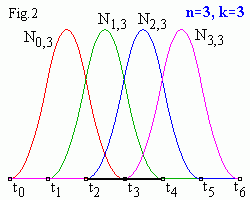# B-spline basis functions

The equation for k-order B-spline with n+1 control points (P0 , P1 , ... , Pn ) is
P(t) = ∑i=0,n Ni,k(t) Pi ,     tk-1 <= t <= tn+1 .
In a B-spline each control point is associated with a basis function Ni,k which is given by the recurrence relations (see Bspline.java)
Ni,k(t) = Ni,k-1(t) (t - ti)/(ti+k-1 - ti) + Ni+1,k-1(t) (ti+k - t)/(ti+k - ti+1) ,
Ni,1 = {1   if   ti <= t <= ti+1 ,    0   otherwise }

Ni,k is a polynomial of order k (degree k-1) on each interval ti < t < ti+1. k must be at least 2 (linear) and can be not more, than n+1 (the number of control points). A knot vector (t0 , t1 , ... , tn+k) must be specified. Across the knots basis functions are C k-2 continuous.Corresponding iterations scheme for cubic (k = 4) basis functions is shown in Fig.1 . You see, that for a given t value only k basis functions are non zero, therefore B-spline depends on k nearest control points at any point t.

B-spline basis functions as like as Bezier ones are nonnegative Ni,k >= 0 and have "partition of unity" property
i=0,n Ni,k(t) = 1,     tk-1 < t < tn+1
therefore
0 <= Ni,k <= 1.
As since Ni,k = 0 for t <= ti or t >= ti+k therefore a control point Pi influences the curve only for ti < t < ti+k.

# Knot vectors

The shapes of the Ni,k basis functions are determined entirely by the relative spacing between the knots (t0 , t1 , ... , tn+k). Scaling or translating the knot vector has no effect on shapes of basis functions and B-spline. Knot vectors are generally: uniform, open uniform and non-uniform.
Uniform knot vectors are the vectors for which   ti+1 - ti = const,   e.g. [0,1,2,3,4,5].
Open Uniform knot vectors are uniform knot vectors which have k-equal knot values at each end:
ti = t0 ,   i < k
ti+1 - ti = const ,   k-1 <= i < n+1
ti = tk+n ,   i >= n+1

e.g. [0,0,0,1,2,3,4,4,4]   (k=3, n=5).
Non-uniform knot vectors. This is the general case, the only constraint is the standard ti <= ti+1 .

# The main properties of B-splines

• composed of (n-k+2) Bezier curves of k-order joined Ck-2 continuously at knot values (t0 , t1 , ... , tn+k)
• each point affected by k control points
• each control point affected k segments
• inside convex hull
• affine invariance
• uniform B-splines don't interpolate deBoor control points (P0 , P1 , ... , Pn )

# Uniform B-splines

The principle thing to note about the uniform basis functions is that, for a given order k, they are simply shifted copies of one another (because all the knots are equispaced). You can see below, that increasing the order k increases smoothness of a curve and tends to move the curve farther from its control polygon.

Linear B-spline (n = 3, k = 2)

Interactive B-spline applet
Drag the mouse to move a nearest control point (a small blue square in the left window) or knot (a small black square in the right window). In the right applet window you see basis polynomials. Move a knot to see how it influences on spline shape and basis functions. B-spline curve is composed of (n-k+2) segments painted in different colors. Corresponding t intervals (in the right window) are painted in the same colors.

Quadratic B-spline (n = 3, k = 3)

Cubic B-spline (n = 3, k = 4)

Closed curves (n = 5, k = 4)

To make a Ck-2 continuous closed loop you need only, that the last k - 1 control points repeat the first k - 1 ones, i.e. [P0 , P1 , P2 , P0 , P1 , P2] for n = 5, k = 4 (in this applet the last 3 points are displaced a bit to make them visible).

Contents   Previous: Building cubic B-spline   Next: Nonuniform B-splines
updated 8 August 2001